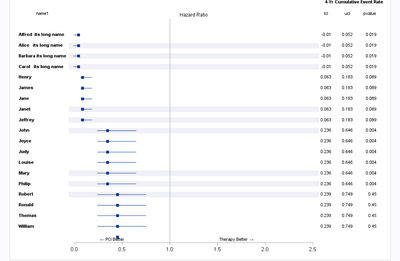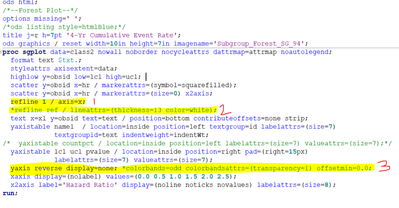## Removing the colorbands from forestplot PROC SGPLOT

I am having the difficulty to to remove the transparent color bands. I tried removing the option colorbands, it did not worked me. what I am doing wrong? is it because of "ods listing style= htmlblue" ? thanks in advance.``````data class ;
set sashelp.class ;
obsid=_n_;

if _n_ <=4 then do;
*Creating lcl negative values  and affecting the x axis in macro;
HR=0.042;
lcl=HR-0.052;
ucl=HR+0.01;
name1 = name||"its long name";
pvalue=0.019;

end;
else if _n_ >=5 and _n_<=9 then do;
HR=0.083;
lcl=HR-0.02;
ucl=HR+0.1;
name1=name;
pvalue=0.089;

end;

else if _n_ >=10 and _n_<=15 then do;
HR=0.346;
lcl=HR-0.11;
ucl=HR+0.3;
name1=name;
pvalue=0.004;

end;

else   do;
HR=0.449;
lcl=HR-0.21;
ucl=HR+0.3;
name1=name;
pvalue=0.45;
end;

Groupid=1;
id= groupid;
keep  hr ucl name1 lcl pvalue groupid obsid id;
run;

/*ods html;*/
/*proc print;run;*/
/*ods html close;*/

/*--Set indent weight, add insets and horizontal bands--*/
data class2;
set class nobs=n end=last;
length text \$20;
val=mod(_N_-1, 6);
if val eq 1 or val eq 2 or val eq 3 then ref=obsid;

/*--Separate Subgroup headers and obs into separate columns--*/
indentwt=1;
if id=1 then indentWt=0;

output;
if last then do;
call missing (name1, pvalue, ucl, lcl, groupid, obsid);
obsid=n+1;
xl=0.4; yl=n+1; text='P'; output;;
xl=1.7; yl=n+1; text='T'; output;
end;
/*   if count ne .;*/
run;

/*--Define Format with Unicode for the left and right arrows--*/
proc format;;
value \$txt
"T" = "Therapy Better (*ESC*){Unicode '2192'x}"
"P" = "(*ESC*){Unicode '2190'x} PCI Better";
run;

/*--Attribute maps for Subgroup Test attributes--*/
data attrmap;
length textweight \$10;
id='text'; value='1'; textcolor='Black'; textsize=7; textweight='bold'; output;
id='text'; value='2'; textcolor='Black'; textsize=5; textweight='normal'; output;
run;
ods html;
/*--Forest Plot--*/
options missing=' ';
/*ods listing style=htmlBlue;*/
title j=r h=7pt '4-Yr Cumulative Event Rate';
ods graphics / reset width=5in height=3in imagename='Subgroup_Forest_SG_94';
proc sgplot data=class2 nowall noborder nocycleattrs dattrmap=attrmap noautolegend;
format text \$txt.;
styleattrs axisextent=data;
refline ref / lineattrs=(thickness=13 color=cxf0f0f7);
highlow y=obsid low=lcl high=ucl;
scatter y=obsid x=hr / markerattrs=(symbol=squarefilled);
scatter y=obsid x=hr / markerattrs=(size=0) x2axis;
refline 1 / axis=x;
text x=xl y=obsid text=text / position=bottom contributeoffsets=none strip;
yaxistable name1  / location=inside position=left textgroup=id labelattrs=(size=7)
textgroupid=text indentweight=indentWt;
/*  yaxistable countpct / location=inside position=left labelattrs=(size=7) valueattrs=(size=7);*/
yaxistable lcl ucl pvalue / location=inside position=right pad=(right=15px)
labelattrs=(size=7) valueattrs=(size=7);
yaxis reverse display=none; *colorbands=odd colorbandsattrs=(transparency=1) offsetmin=0.0;
xaxis display=(nolabel) values=(0.0 0.5 1.0 1.5 2.0 2.5);
x2axis label='Hazard Ratio' display=(noline noticks novalues) labelattrs=(size=8);
run;

``````
1 ACCEPTED SOLUTION

Accepted Solutions

## Re: Removing the colorbands from forestplot PROC SGPLOT

Comment out or remove this line:

```  refline ref / lineattrs=(thickness=13 color=cxf0f0f7);
```

and those shaded areas go away.

Borrowed code without understanding what the REF variable was used for?

Or attempting to do something else that did not work?

6 REPLIES 6Jay54
Meteorite | Level 14

## Re: Removing the colorbands from forestplot PROC SGPLOT

Maybe remove the reflines.

## Re: Removing the colorbands from forestplot PROC SGPLOT

It worked. Thank you.

## Re: Removing the colorbands from forestplot PROC SGPLOT

Comment out or remove this line:

```  refline ref / lineattrs=(thickness=13 color=cxf0f0f7);
```

and those shaded areas go away.

Borrowed code without understanding what the REF variable was used for?

Or attempting to do something else that did not work?

## Re: Removing the colorbands from forestplot PROC SGPLOT

Thanks @ballardw  I am new at sgplot and , yes it's borrowed code.  I got confused between two refline statements and colorbands options in Y axis, So I was not sure which one causing the  bands, I thought it was like graph wall display.

Thanks you @Jay54  and you for pinpointing the  statement. It worked for me ( I tried removing the refline statement or changing the lineattrs color= white. Can you guys please spare some time what these statement/ or options do in quick a way.As per numbering in Image:

1. I was expecting that it creates the solid reference line at 1 on x axis. How I can change the color /pattern of this line if I want give line attrs  options on this statement?

2. Commented code: it controls the attributes of the above reference line such as color, thickness, or pattern. But Look like I am wrong😣

3. What is the option color bands do in general? I expected the background color came from this option where the transparency of the color, and even and odd location can be changed here. But I am wrong again. here.

## Re: Removing the colorbands from forestplot PROC SGPLOT

Suggest that you read the documentation from the syntax for options. That way you know where to look them up when you need them.

Your code does create a solid reference line at 1 on the X axis. Why do you think it doesn't.

Each REF statement is separate. If want multiple options applied to one reference line, or group, then all the options go on one statement. Your 'Ref Ref ' statement had only some reference lines because of the way that you assigned the values to the variable ref. Why they are done that way was one of the reasons I asked if this was borrowed code as you should have expected something there.  Color, pattern and thickness are the only options for LINEATTRS. Transparency is a separate option.

COLORBANDS only affects discrete axis. So since your Y axis is not explicitly set to discrete such options aren't typically applied. The option would alternate background color at the midpoints between tick mark values.

## Re: Removing the colorbands from forestplot PROC SGPLOT

I understood better now. Thanks for the document. I definitely need to read that.

Discussion stats
• 6 replies
• 465 views
• 4 likes
• 3 in conversation